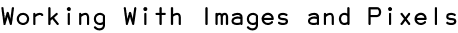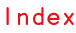RSS

Using Scriptographer you can easily work with the colors of individual pixels of images and average colors of areas within images.

When coding with Scriptographer we refer to an image in an Illustrator document as a Raster.

## Finding the first selected image in a document

When working with images, we usually want the user to select an image and then execute a script which does something with that image. If the script was executed without first selecting an image, we show a dialog which asks the user to first select an image.

```// find all selected images in the document
var rasters = document.getItems({
type: Raster,
selected: true
});

// check if any images were selected
if(rasters.length > 0) {
// get the first raster in the rasters array
var raster = rasters;

// do things with the raster here
// ...

} else {
}
```

## Colors of Pixels

To find the color of a specific pixel in a raster, we use raster.getPixel(x, y) and pass it the x and y offset of the pixel we want to look at. Depending on the color space of the raster, the raster.getPixel(x, y) function returns an RGBColor, CMYKColor or GrayColor.

The following script loops through the pixels of the selected raster and creates a circle shaped path for each of them and fills them with the color of the pixels.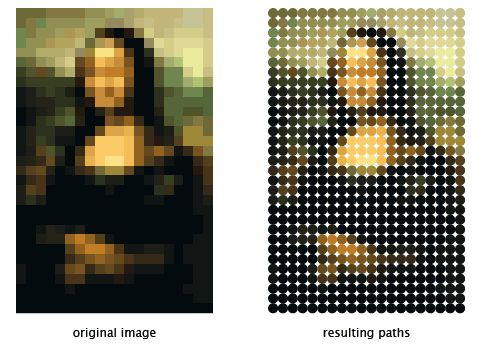The following code snippet should be pasted after line 13 in the script at the top of the page which finds the selected raster in your document.

```var gridSize = 10;

for(var y = 0; y < raster.height; y++) {
for(var x = 0; x < raster.width; x++) {
var color = raster.getPixel(x, y);
var position = new Point(x, y) * gridSize;
var path = new Path.Circle(position, gridSize / 2);
path.fillColor = color;
}
}
```

Now lets go through the script line by line.

First we define a variable which contains the size of our grid:

```var gridSize = 10;
```

We could put this value directly into the place where we use it later on (line 5), but by naming it we can easily see what the value is meant for. It is considered good practice to place these types of variables at the top of a script, to give anyone looking into the script an idea of things they could change to get a different outcome.

Next, we want to loop through each pixel of the raster. The raster.getPixel(x, y) needs an x and y value to be able to locate a pixel in the raster. To do this, we need to make two loops.

The first loop runs through the rows of the raster (raster.height). The y variable starts at 0 and every time the loop is executed we increment it with 1, until it reaches the value of raster.height.

```for(var y = 0; y < raster.height; y++) {
// this code block is executed raster.height times
}
```

Within the row loop we have another loop that runs through all the columns, giving us two variables: y describes the row that we're on and x describes the column.

```for(var y = 0; y < raster.height; y++) {
// the following loop is executed for every row
for(var x = 0; x < raster.width; x++) {
// this code block is executed raster.width times
}
}
```

Now lets look at the code we execute for every row/column combination.

First of all, lets find the color in the raster using the x and y variables that we receive from the loops and store it in a variable called color:

```var color = raster.getPixel(x, y);
```

Next we need to find the position where we will make the circle shaped path. To do this, we construct a point using the x and y variables that we receive from the loops and multiply it by the value contained in the gridSize variable:

```var position = new Point(x, y) * gridSize;
```

All right, now we just need to create the path and fill it with the color of the pixel.

We pass the new Path.Circle(center, radius) constructor our position point to position the center point of the circle and we pass it gridSize / 2 as the radius of the circle:

```var path = new Path.Circle(position, gridSize / 2);
```

And we pass the color we found earlier to item.fillColor to fill it with that color:

```path.fillColor = color;
```

## Using Color Channel Values

Instead of directly using the colors returned from raster.getPixel(x, y), we can also use the values of their individual channels to do different things. The color channels of a color contain a value between 0 and 1.

The following script loops through the pixels of the selected raster and creates a grid of circle shaped paths of varying sizes depending on the gray value of their colors.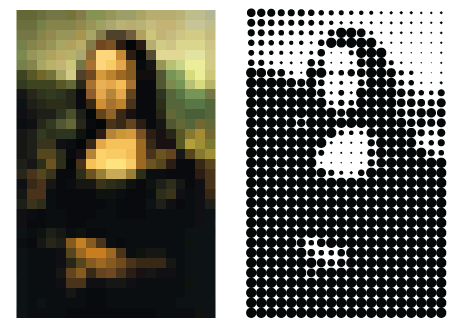The following code snippet should be pasted after line 13 in the script at the top of the page which finds the selected raster in your document.

```var gridSize = 10;

for(var y = 0; y < raster.height; y++) {
for(var x = 0; x < raster.width; x++) {
var color = raster.getPixel(x, y);
var position = new Point(x, y) * gridSize;
var radius = (gridSize / 2) * color.gray;
var path = new Path.Circle(position, radius);
}
}
```

This code is very similar to the example above, but this time we don't fill the path with the color we find when we call Raster.getPixel(x, y). Instead, we look at the grayness of the color, which is a value between 0 (white) and 1 (black). We multiply the radius by this amount to make circles that have a radius ranging from (gridSize / 2) * 1 when the color of the pixel is black to (gridSize / 2) * 0 when the color of the pixel is white:

```var radius = (gridSize / 2) * color.gray;
```

Of course we can use the color.gray value for anything. In the following example we create rectangular shaped paths which we rotate and scale by the amount of gray in the pixels: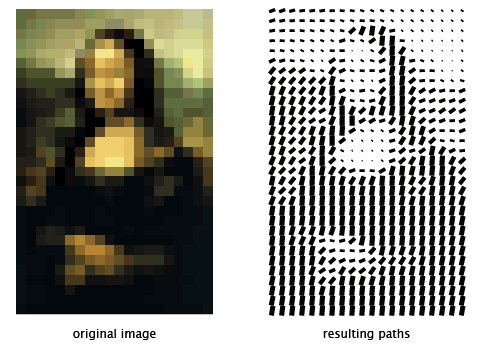The following code snippet should be pasted after line 13 in the script at the top of the page which finds the selected raster in your document.

```var gridSize = 10;
var rectSize = new Size(gridSize / 2, gridSize);

for(var x = 0; x < raster.width; x++) {
for(var y = 0; y < raster.height; y++) {
var color = raster.getPixel(x, y);
var position = new Point(x, y) * gridSize;
var radius = (gridSize / 2) * color.gray;
var path = new Path.Rectangle(position, rectSize);
path.scale(color.gray);
var rotation = 180 * color.gray;
path.rotate(rotation);
}
}
```